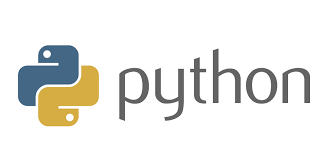# How to Intersect Two Dictionaries in Python

Sometimes you may need to find common elements between two python dictionaries. In this article, we will look at how to find common keys between two python dictionaries. In other words, here is how to intersect two dictionaries in python.

## How to Intersect Two Dictionaries in Python

Let us say you have the following two python dictionaries.

```>>> dict1={1:'one',2:'two',3:'three',4:'four'}
>>> dict2={3:'three',4:'four',5:'five',6:'six'}```

We will look at two different ways to find common keys between two dictionaries.

## Intersect dictionaries using dict comprehension

You can easily get intersection of keys using dict comprehension as shown below.

```>>> common_dict = {x:dict1[x] for x in dict1 if x in dict2}
>>> common_dict
{3: 'three', 4: 'four'}```

In the above dict comprehension we basically loop through dict1 elements and check whether they exist in dict2.

Also read : How to Raise Exception in Python

## Intersect dictionaries using & operator

Python 3 onwards, you also get a ‘&’ operator that allows you to easily intersect two python dictionaries.

```>>> common_dict = dict(dict1.items() & dict2.items())
>>> common_dict
{3: 'three', 4: 'four'} ```

In case of python 2 (<3), you will have to convert the keys of these two dicts into sets yourself and then get the intersection using & operator.

```>>> dict1_keys=set(dict1.keys())
>>> dict2_keys=set(dict2.keys())
>>> common_keys=dict1_keys & dict2_keys
>>> common_keys
set([3, 4])```

Once have the common keys, you need to get their associated elements from either of the dictionaries.

```>>> common_dict={x:dict1[x] for x in common_keys }
>>> common_dict
{3: 'three', 4: 'four'}```

Please note, in both the above methods, we have assumed that the associated values are the same in case the keys are the same. For example, 3:’three’ in both the dictionaries. If they are different (e.g 3:’three’ in dict1 and 3:’thirty’ in dict2) then you need to make a choice which value to pick in the intersection, and modify your dict comprehension accordingly.

That’s it. As you can see, it is easy to intersect two dictionaries. The first method using dict comprehensions works on all Python versions. The second method using & operator works easily only if you have python 3+. Otherwise, it is tedious to get common keys and then create the intersection dictionary.

So it is recommended to use the first method to intersect two dictionaries.

Also read : How to Extract data from JSON file in Python